## Written addition of money (pg 1)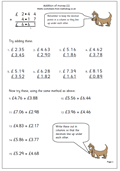Once children are familiar with the standard method of addition for 3 digits they can be introduced to addition of decimals. One of the best ways to do this is by adding money.  On this page the first eight questions have been written out in the correct way, but the next seven will also need to be written out using the same method. The key here is to keep the decimal point in line as the numbers in later addition of decimals may not necessarily all have two digits after the decimal point. Also, don’t forget the £ sign in the answer.

## Coming soon: Tally charts and bar charts and addition.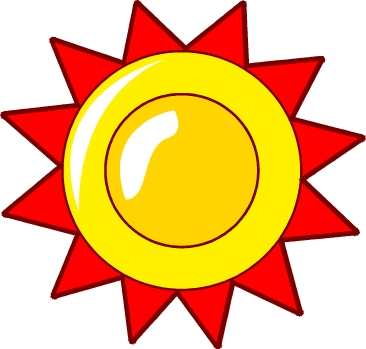This week we have two worksheets on data handling. One looks at interpreting a tally chart on illnesses which people had when they were children. As well as answering questions which can be worked out mathematically it is important to try and use the data to draw some conclusions. So it is a good idea to discuss the results shown and think whether they show a trend or are they saying anything significant about the world we live in e.g.

The second is a bar chart of a type which children may not have come across before. It shows the frequency of events; in this case the frequency of the number of goals scored by ‘The Albion’. The term ‘mode’ is used, which means the most common as well as other terms such as ‘maximum’. These types of graph are quite tricky to interpret and is most suited to Year 5.

We also have another page on standard addition with money – don’t forget those decimal points!

## Year 3 Maths worksheet: Money problems 2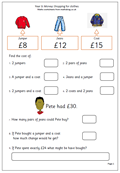The second in our series of worksheets on money for year 3 children. This page looks at addition of whole pounds. Again, the questions should be answered mentally, perhaps with the help of jottings.

The last 3 questions are slightly more complex and involve a higher level of  problem solving skills. It is important that children become confident with dealing with money as most of their real life calculations will have to be done without the help of pencil and paper or calculator.

Money: Shopping for clothes (pg 1)

## Resource of the Week: Metric worksheets for Year 4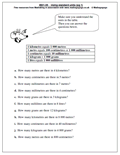We have been using metric measurement for a long time now yet many children still seem confused when measuring, whether it is distance, weight or capacity.

There are a few simple facts that children need to know, including:

1 kilometre equals 1000 metres

1 kilogram = 1000 grams

1 litre equals 1000 millilitres

Not too tricky and much easier than the old imperial unts where 1760 yards equals one mile!!

There are a number of excellent metric worksheets for Year 4, thanks to Mathsgogogo and MathSphere for allowing me to ‘borrow’ them.

Year 4 metric worksheets

## Standard method of addition of 4-digits (2)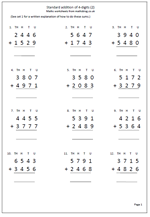Here is another maths worksheet of addition of two 4-digit numbers. To be successful with these children need to have a very good knowledge of number bonds up to ten eg know that 6 + 5 is 11. They must also be confident with place value, understanding that each column of numbers needs to be vertically in a line.

A full explanation of this method can be found on the first of the adding two 4-digit numbers worksheet.

Standard addition of 4-digits (pg 2)

## Mental Arithmetic: Subtracting 19 from 2-digit numbers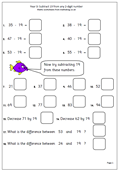This is very similar to the process of adding 19 to a 2-digit number. The easiest way to do this is usually to take twenty and then add one. This mental arithmetic method is much quicker than carrying out the question using pencil and paper methods.If children can grasp these ideas it gives them a great deal of confidence and they can develop their own mental methods of answering other, similar questions; eg subtract 18 by taking 20 and then adding 2, or subtracting 39 by taking forty and adding one.

## Coming Soon: Addition, subtraction and money problems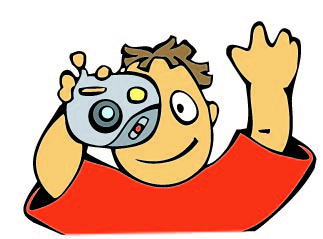Next week we have a page explaining how to subtract 19 from a 2-digit number. The easiest way to do this is usually to take twenty and then add one and is much quicker than using pencil and paper methods. We will also be publishing the second of our adding two 4-digit numbers using the standard written method. Also coming soon is a page of money problems most suited to Year 3 children.

With just a week or two left of the long summer holiday it’s probably not easy to think about maths, but just 10-15 minutes in the evening can just clear the cobwebs, ready for a new year!

## Year 5 maths worksheet: add decimals mentally (2)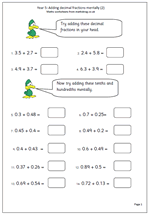Another in our series of adding decimals mentally, suitable for year 5 children. A good understanding of place value and decimals is needed for this, especially for the second set of questions.

Look out for a common error. For example: 0.3 + 0.48 answered as 0.51. In this case the 0.3 has been thought of as 0.03 and added to the hundredths when it should be added to the tenths, so the correct answer is 0.78.

We are looking for quick responses to these types of question. If children are taking a long time over them it might be because they have not learned their addition facts to 10 sufficiently so that they still need to count on to work the answers out.

Further similar pages to this can also be found in our Four Rules section.

Add decimal fractions mentally (pg 2)

## Resource of the Week: Divide by 5 mentally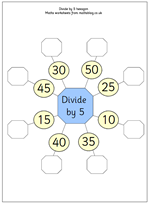Learning tables is considered an essential to maths progress, and indeed, it is vital. But knowing tables is only a starting point: these facts then need to be used. The most obvious use other than to multiply numbers is to do the reverse; to divide numbers. We have a number of resources which look dividing ‘in your head’ including this one on divide by 5 mentally.

Essentially it is the reverse of a times table sheet and highlights that if 4 x 5 = 20 is known then 20 divided by 4 = 5 should come quickly after it.

Mental arithmetic: divide mentally

## Time: Finding lengths of time (2)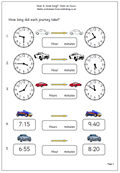This is the second in our time worksheets for Year 3 children. It is quite a bit harder than the first as it deals with hours and minutes rather than just minutes.

With the analogue displays the best way to approach this is to count on the whole hours and make a note if necessary, and then count on the extra minutes, usually in steps of 5 minutes.

With the digital display again work out the hours and then count on. This can be tricky as you may need to stop at the hour before if the minutes on the later time are less than the minutes on the earlier time (sounds complicated but an example would be from 6:55 to 8:20, only count on one whole hour to 7:55).

Finding lengths of time (pg 2)